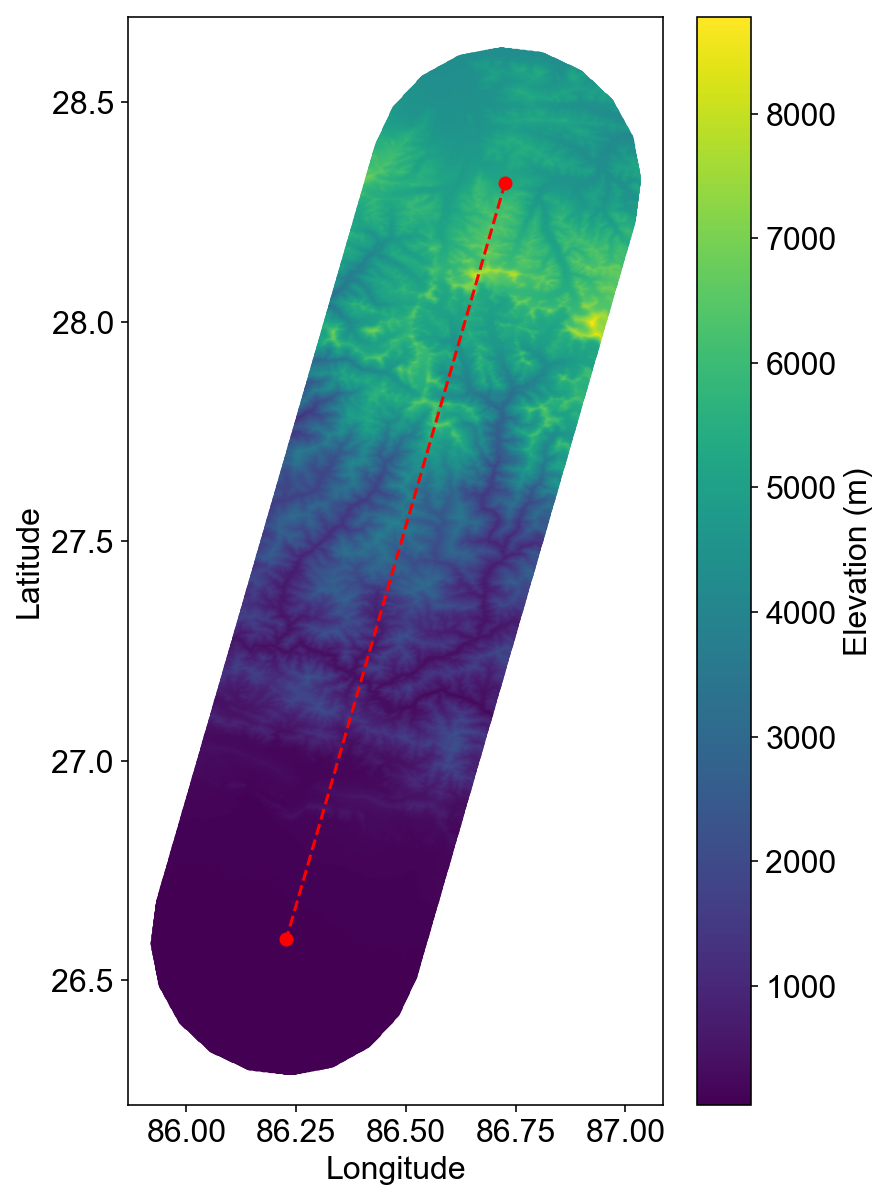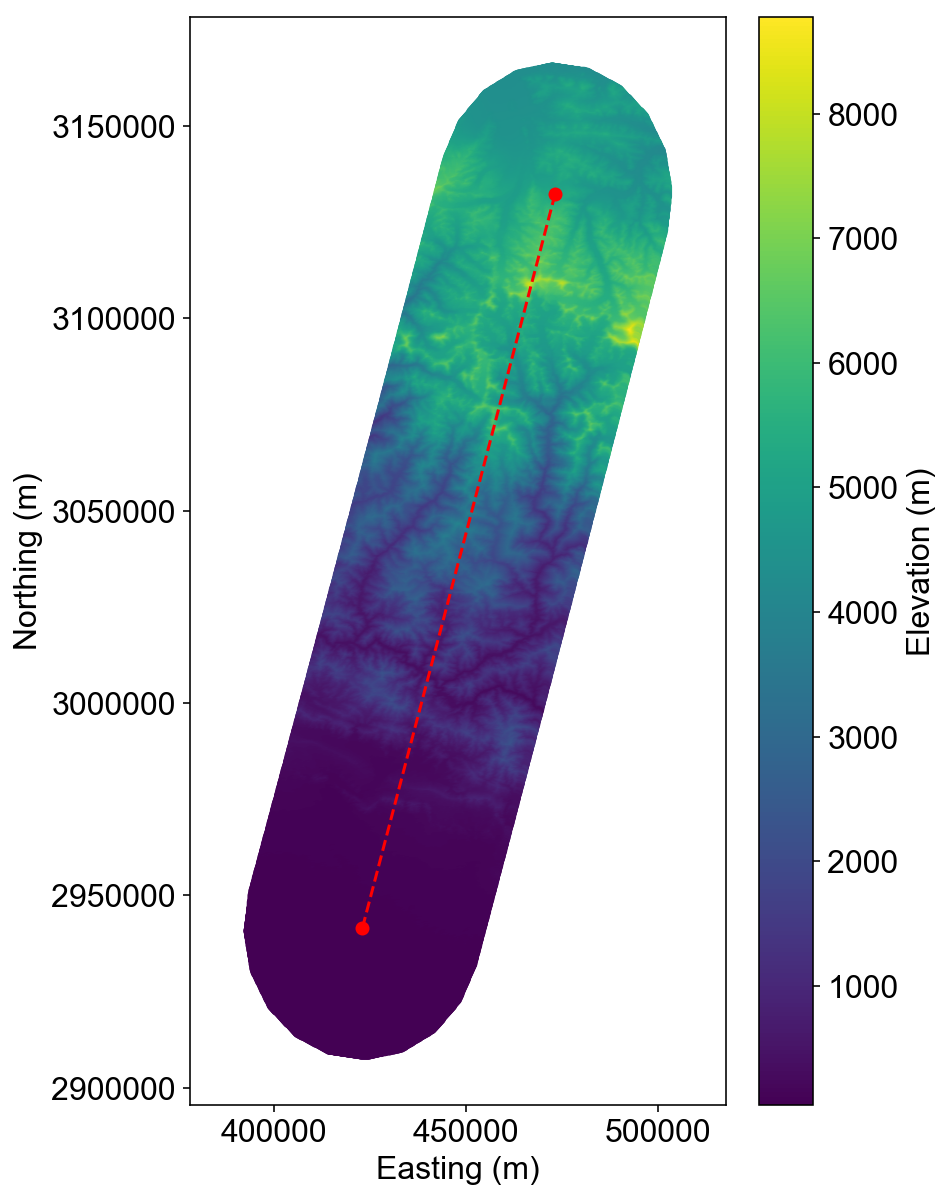Exploring strength and stress relationships in critical wedges with raw topography data through Bayesian inversions

Richard Styron

I received an email a few weeks ago from my friend Sean Gallen asking about Bayesian modeling of critical wedges, in order to really explore the relationship between fault strength and tectonic stress. As it was a far more interesting use of my afternoon than my actual responsibilities (slogging through the paper I was writing), I of course dropped everything and went to work.

Sean was interested in a form of the critical wedge model from Suppe (2007). The full critical wedge equation from Dahlen (1990):

$$\alpha + \beta = \frac{\beta[1-(\rho_f / \rho)] + \mu_b(1-\lambda_b) + S_b / \rho g H} {[1-(\rho_f / \rho)] + 2(1-\lambda) \left[\frac{\sin \phi}{1 - \sin \phi }\right] + C / \rho g H}$$

where $\alpha$ is the wedge surface slope, $\beta$ is the basal thrust (decollement) slope), $\rho$ is rock density, $\rho_f$ is the density of the fluid surrounding the wedge (air or water), $\mu_b$ is the coefficient of friction of the basal thrust, $\lambda$ is the pore fluid pressure in the wedge normalized by depth (i.e. $\rho g H$), $\lambda_b$ is the pore pressure of the decollement, $\phi$ is the angle of internal friction, $S_c$ is the basal cohesion/plasticity, and $C$ is the uniaxial compressive strength of the wedge.

Suppe consolidates the equation by gathering several terms into $F = \mu_b(1-\lambda_b) + S_b / \rho g H$ and $W = 2 ( 1 - \lambda) [\sin \phi / (1 - \sin \phi)] + C / \rho g H$. $F$ represents fault strength and $W$ represents the wedge strength. Since the model is at critical (Coulomb) failure, these terms also represent stresses: $F = \sigma_\tau / \rho g H$ and $W = (\sigma_1 - \sigma_3) / \rho g H$.

This consolidation allows us to simplify the big equation above into a linear relationship between the surface slope $\alpha$ and the decollement slope $\beta$,

$$\alpha = \frac{F}{\left[1 - (\rho_f / \rho) \right] + W} - \frac{W}{\left[1-(\rho_f / \rho)\right]+W}\beta$$

which is of the form $y = m x + b$, i.e. a line.

Suppe uses this linear relationship with sets of ($\alpha$, $\beta$) measurements from a few active wedges to estimate $F$ and $W$ through linear regression. This is a good approach if you have multiple sets of $\alpha$ and $\beta$ measurements for a single wedge that have a bit of variation and a linear relationship.

However, this isn't exactly our problem. We are more interested in a single transect (with one value for $\alpha$ and $\beta$) and finding out how $F$ and $W$ covary. Furthermore, Sean has insightfully noted that calculating $\alpha$ directly from DEM-derived slope data is problematic, because you either have to filter/smooth the topography data or you just get really noisy $\alpha$ measurements. However, you can instead take a value for $\alpha$ and then predict the elevation at a point in the wedge, and compare that to the real elevation, which eliminates a lot of the messiness.

So we're going to work on that. We could definitely do this for multiple transects in a wedge, especially if we have good data from somewhere on the basal detachment geometry, and really try to pin down $F$ and $W$ as Suppe did. But that's a blog post for a different day.

If I had any (regular?) readers of this blog, these fictional persons would surely realize by now that we're going to do all of this with Python in a Jupyter notebook. The notebook is available here and the underlying data are here and here. You will need to change the paths specified below to wherever you download the files to run the notebook.

In :
%matplotlib inline
%config InlineBackend.figure_format = 'retina'
In :
import json
import time
import numpy as np
from scipy.stats.mstats import gmean
import matplotlib.pyplot as plt
import pandas as pd
import pyproj as pj
In :
from multiprocessing import Pool
In :
import matplotlib
matplotlib.rcParams.update({'font.size': 16})

First we're going to get the data.

The transect is a line normal to the Himalayan wedge, running through some locally high topography. Then, I used a 0.3° buffer around it to extract points from the 250 m SRTM dataset. We will use these points to find the x axis for the analysis as well.

In :
with open('../data/transect_line.geojson', 'r') as f:
transect_coords = transect_pts_gj['features']['geometry']['coordinates']

transect_pts = pd.DataFrame(data=transect_coords,
columns=['lon', 'lat'],
index=['p1', 'p2'])
In :
transect_pts # these are the end points of the line.
Out:
lon lat
p1 86.726076 28.316242
p2 86.227448 26.592723
In :
# convert to utm45

utm45 = pj.Proj(init='epsg:32645')
wgs84 = pj.Proj(init='epsg:4326')

transect_pts['easting'], transect_pts['northing'] = pj.transform(wgs84, utm45,
transect_pts['lon'].values,
transect_pts['lat'].values)
In :
transect_pts
Out:
lon lat easting northing
p1 86.726076 28.316242 473146.100052 3.132265e+06
p2 86.227448 26.592723 423075.501911 2.941559e+06

Now we're going to read in a topography dataset, in .csv format, with Pandas

In :
names=['lon', 'lat', 'elev'])

Look at the end of the data file:

In :
topo_pts.tail()
Out:
lon lat elev
312103 86.239065 26.294209 57
312104 86.241148 26.294209 55
312105 86.243232 26.294209 54
312106 86.245315 26.294209 56
312107 86.247398 26.294209 53

Now let's make a little plot:

In :
plt.figure(figsize=(6,10))
plt.scatter(topo_pts.lon, topo_pts.lat,
c=topo_pts.elev,
lw=0, s=20)
plt.plot(transect_pts['lon'], transect_pts['lat'], 'ro--')
plt.xlabel('Longitude')
plt.ylabel('Latitude')
plt.colorbar(label='Elevation (m)')
plt.axis('equal')
plt.show()In :
# Project these points into UTM 45N
topo_pts['easting'], topo_pts['northing'] = pj.transform(wgs84, utm45,
topo_pts.lon.values,
topo_pts.lat.values)
In :
plt.figure(figsize=(6,10))
plt.scatter(topo_pts.easting, topo_pts.northing,
c=topo_pts.elev,
lw=0, s=20)

plt.plot(transect_pts['easting'], transect_pts['northing'], 'ro--')

plt.colorbar(label='Elevation (m)')
plt.xlabel('Easting (m)')
plt.ylabel('Northing (m)')
plt.axis('equal')
plt.show()Rotating points to transect coordinates¶

The critical wedge model that we're using is in two dimensions, with the x-axis along the transect (i.e. the red line in the plots above) and the y-axis as elevation. So we need to rotate and translate all of the points into this coordinate system, which will smash all of the lateral variation; the lateral variation then becomes the y-scatter in the data.

First we find the east and north coordinates in meters relative to the southern transect point, p2, which will be the new origin.

In :
topo_pts['rel_east'] = topo_pts['easting'] - transect_pts.loc['p2','easting']
topo_pts['rel_north'] = topo_pts['northing'] - transect_pts.loc['p2','northing']
In :
plt.figure(figsize=(6,10))
plt.scatter(topo_pts.rel_east, topo_pts.rel_north,
c=topo_pts.elev,
lw=0, s=20)

plt.colorbar(label='Elevation (m)')
plt.xlabel('east (m)')
plt.ylabel('north (m)')
plt.axis('equal')
plt.show()